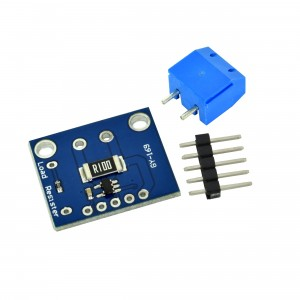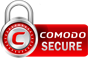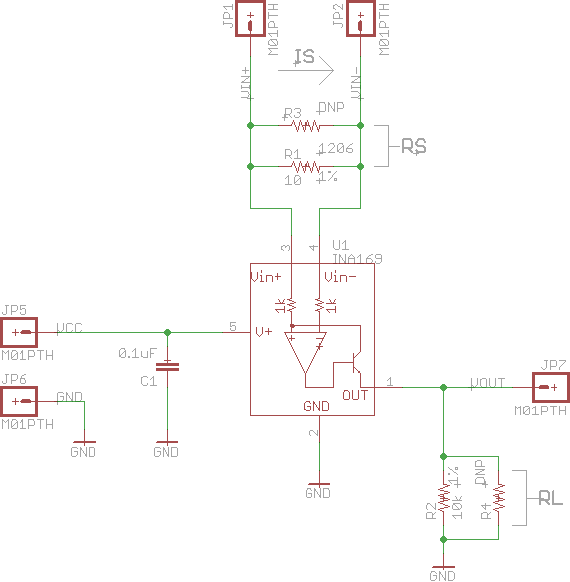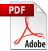# INA169 Current Sensor ModuleINA169 current sensor is an ideal way to measure current in various projects, easily used with Arduino boards.

SKU: OTH531226414645,WUM536733044850

Price: 4.22 \$

Product in stockSSL Certificate
Quantity

# Overview

This module can solve the problems of monitoring the current. Instead of using multi-meters, INA169 can be used for both image and output DC current measurement analog offered. Analog output makes this module ideal for monitoring current.

Resistance measurement can operate at 2W DC, so it can measure up to + 5A. The output is a current passing through a 10k resistor, so the output voltage is 1V on Amp. It can change the resistance value by cutting resistance through hole resistors. If you add a resistance of 20K, you will get 2V per Amp and a resistance of 5K will get 0.5V per Amp.

A 5-pin module can be attached to your project with ease. It also has a 3.5 mm terminals for connecting / disconnecting.

This module is very easy to use, it is only necessary to connect a power supply between 2.7 V to 60 V, connect V + to V- from the positive supply and ground. Then measure the output using a multi meter.

# Specifications

• Resistance: 0.1 ohm 1% 2 W
• Supply Voltage: 2.7 V to 60 V

# Arduino code example

/*
Hardware connections:

Uno Pin    INA169 Board    Function

+5V        VCC             Power supply
GND        GND             Ground
A0         VOUT            Analog voltage measurement

VIN+ and VIN- need to be connected inline with the positive
DC power rail of a load (e.g. an Arduino, an LED, etc.).

*/

// Constants
const int SENSOR_PIN = A0;  // Input pin for measuring Vout
const int RS = 10;          // Shunt resistor value (in ohms)
const int VOLTAGE_REF = 5;  // Reference voltage for analog read

// Global Variables
float sensorValue;   // Variable to store value from analog read
float current;       // Calculated current value

void setup() {

// Initialize serial monitor
Serial.begin(9600);

}

void loop() {

// Read a value from the INA169 board

// Remap the ADC value into a voltage number (5V reference)
sensorValue = (sensorValue * VOLTAGE_REF) / 1023;

// Follow the equation given by the INA169 datasheet to
// determine the current flowing through RS. Assume RL = 10k
// Is = (Vout x 1k) / (RS x RL)
current = sensorValue / (10 * RS);

// Output value (in amps) to the serial monitor to 3 decimal
// places
Serial.print(current, 3);
Serial.println(" A");

// Delay program for a few milliseconds
delay(500);

}For more details check the attached datasheet.

•ina169.pdf